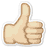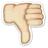depts.gpc.edu

No badges were found

Definitions (222)

# 1

00# mol math glossary

large; text-align:center; font-weight:bold"> A
 Source: depts.gpc.edu

# 2

00# a abacus

an oriental (far east) counting device and calculator. abscissa
 Source: depts.gpc.edu

# 3

00# absolute value

The symbol for absolute value is usually a pair of vertical lines containing the number. The symbolism |4| is read as "The absolute value of four". The absolute value of a real number is its distance from zero, so |4| = |-4| = 4 ... which is the distance of the number from zero. The positive value. acceleration
 Source: depts.gpc.edu

# 4

00# acute angle

a positive angle that measures less than 90 degrees ... an angle in the range of 0 degrees to 90 degrees. acute triangle
 Source: depts.gpc.edu

# 5

00The number zero is called the additive identity because when you add it to a number, N, the result you get is the same number, N ... 0 + 5 = 5. additive inverse
 Source: depts.gpc.edu

# 6

00two angles that share both a common side and vertex. affine cipher :
 Source: depts.gpc.edu

# 7

00# alternate exterior angles

Angles located outside a set of parallel lines and on opposite sides of the transversal. alternate interior angles:
 Source: depts.gpc.edu

# 8

00# altitude

the perpendicular distance measured from the base of a figure to the highest point of the figure. amplitude
 Source: depts.gpc.edu

# 9

00# angle

the union of two rays which have a common endpoint (starting point). antecedent
 Source: depts.gpc.edu

# 10

00# antiderivative

the antiderivative of a function, f(x), is a function, F(x), whose derivative is f(x). Also called the indefinite integral. apothem
 Source: depts.gpc.edu